### 6.1 Synopsis

The aim of the AcousticSolver is to predict acoustic wave propagation. Through the application of a splitting technique, the flow-induced acoustic field is totally decoupled from the underlying hydrodynamic field.

#### 6.1.1 Linearized Euler Equations

The Linearized Euler Equations (LEE) are obtained by linearizing the Euler Equations about a mean flow state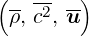. Hence, they describe the evolution of perturbations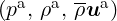around this state. In conservative form, the LEE are given as: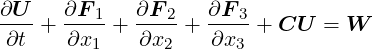(6.1)

with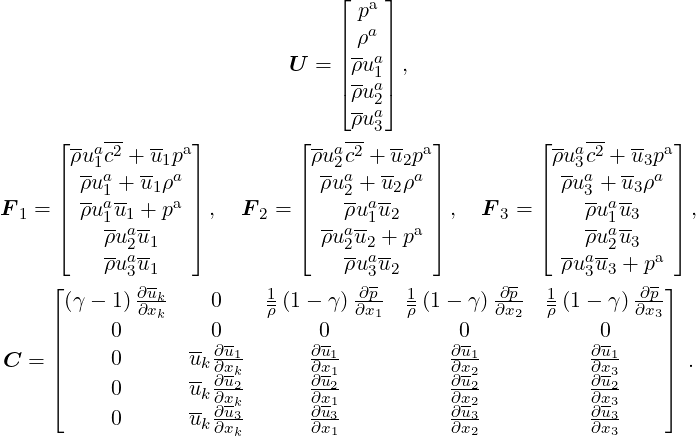(6.2)(6.3)(6.4)
By default, the source term vector W is zero and has to be specified by an appropriate forcing.

#### 6.1.2 Acoustic Perturbation Equations

The acoustic perturbation equations (APE-1/APE-4) proposed by Ewert and Schroeder  assure stable aeroacoustic simulations. These equations are similar to the LEE, but account for acoustic perturbations exclusively. The AcousticSolver implements the APE-1/4 type operator: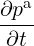+ c2∇⋅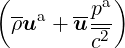=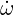c (6.5a)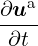+ ∇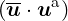+ ∇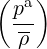=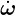m , (6.5b)

where (u,c2,ρ) represents the base flow and (ua,pa) the acoustic perturbations. Similar to the LEE, the acoustic source termsc and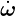m are by default zero and must be specified e.g. by an appropriate forcing. This way, e.g. the APE-1, APE-4  or revised APE equations  can be obtained. Expressed as hyperbolic conservation law, the APE-1/4 operator reads: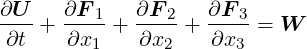(6.6)

with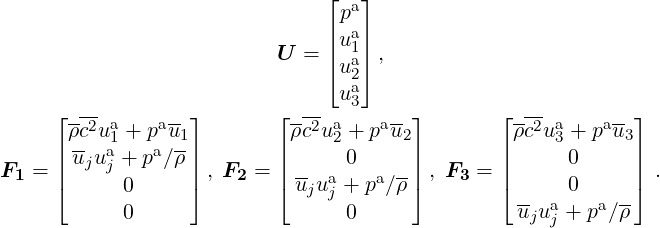(6.7)(6.8)